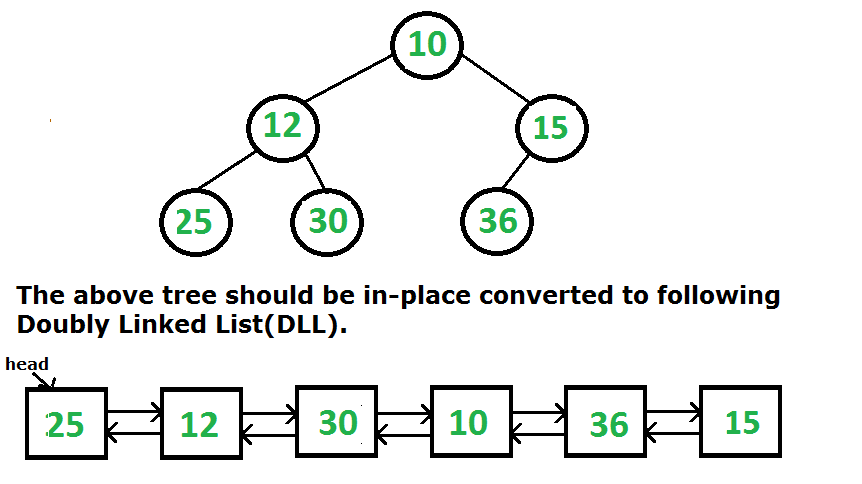Convert a given Binary Tree to Doubly Linked List | Set 3

• Difficulty Level : Medium
• Last Updated : 02 Dec, 2021

Given a Binary Tree (BT), convert it to a Doubly Linked List(DLL) In-Place. The left and right pointers in nodes are to be used as previous and next pointers respectively in converted DLL. The order of nodes in DLL must be the same as in Inorder for the given Binary Tree. The first node of Inorder traversal (leftmost node in BT) must be the head node of the DLL.The following two different solutions have been discussed for this problem.
Convert a given Binary Tree to Doubly Linked List | Set 1
Convert a given Binary Tree to Doubly Linked List | Set 2

In this post, a third solution is discussed which seems to be the simplest of all. The idea is to do in order traversal of the binary tree. While doing inorder traversal, keep track of the previously visited node in a variable, say prev. For every visited node, make it next to the prev and previous of this node as prev.
Thanks to rahul, wishall and all other readers for their useful comments on the above two posts.

The following is the implementation of this solution.

Javascript



Output:

25 12 30 10 36 15

Note that the use of static variables like above is not a recommended practice (we have used static for simplicity). Imagine a situation where the same function is called for two or more trees. The old value of prev would be used in the next call for a different tree. To avoid such problems, we can use a double-pointer or reference to a pointer.
Time Complexity: The above program does a simple inorder traversal, so time complexity is O(n) where n is the number of nodes in given binary tree.

The below code is the intuitive iterative implementation of the above inorder traversal approach (inspired from this video) –

C++

 Node * bToDLL(Node *root){    stack> s;    s.push({root, 0});    vector res;    bool flag = true;    Node* head = NULL;    Node* prev = NULL;    while(!s.empty()) {        auto x = s.top();        Node* t = x.first;        int state = x.second;        s.pop();        if(state == 3 or t == NULL) continue;        s.push({t, state+1});        if(state == 0) s.push({t->left, 0});        else if(state == 1) {            if(prev) prev->right = t;            t->left = prev;            prev = t;            if(flag) {                head = t;                flag = false;            }        }        else if(state == 2) s.push({t->right, 0});    }    return head;}

Time complexity: O(N)

Space complexity: O(N)